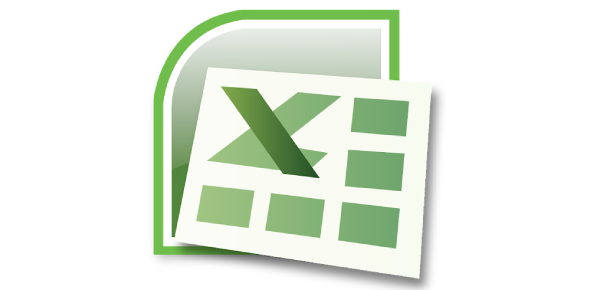# Microsoft Excel 2007 Quiz: Exam!

76 Questions | Total Attempts: 1649SettingsAre you familiar with Microsoft Excel 2007? Would you like to attempt to answer these questions? One of the significant improvements in Microsoft Excel 2007 is the level of resting that can be achieved. Comparatively speaking, Excel 2003 only allowed seven resting functions, while Excel 2007 permits up to 64. Another significant difference is that Excel 2007 provides more columns and rows if you want to learn more and complete this quiz.

• 1.
What is a workbook made up of?
• 2.
The ____________ displays the cell reference of the active cell.
• 3.
A(n) ________ function is conditional.
• 4.
In pie charts with legends, it's best to make the slice colors as similar as possible.
• A.

True

• B.

False

• 5.
Although 3D Charts are visually attractive, they can obscure the relationship between the values in the chart by making it difficult to see which slice is larger.
• A.

True

• B.

False

• 6.
You can rotate a 3D chart in only two directions: horizontally along the x-axis and vertically along the y-axis.
• A.

True

• B.

False

• 7.
In excel, charts do not remain linked or connected to their data sources if they appear in different worksheets.
• A.

True

• B.

False

• 8.
Excel has a built-in format to display 4 digit year values, so you do not need to create one.
• A.

True

• B.

False

• 9.
One challenge of nesting functions is to make sure that you include all of the parentheses.
• A.

True

• B.

False

• 10.
A "mixed reference" locks one part of the cell reference while the other part can change.
• A.

True

• B.

False

• 11.
To enter a mixed reference, type \$ after either the row or column reference.
• A.

True

• B.

False

• 12.
If a formula contains several functions, Excel starts with the outermost function and then moves inward.
• A.

True

• B.

False

• 13.
As you begin to type a function name within a formula into a cell, a list of functions that begin with the letters you typed appears.
• A.

True

• B.

False

• 14.
Pie charts are most effective with ___________ or fewer slices.
• 15.
This is a collection of one or more data series.
• 16.
This compares three sets of values in a 3D chart.
• 17.
This compares a collection of values from several different data sets.
• 18.
This compares relative values of different categories to the whole; similar to the pie chart except that it can display multiple sets of data.
• 19.
This compares value from different categories; often used to show trends and changes over time.
• 20.
What is a range of values that is plotted as a single unit on the chart.
• 21.
What displays values in different categories; column chart on its side.
• 22.
What uses vertical rectangles to compare values across categories.
• 23.
In "SUM(number1 [,number2 ,number3 ...])", number 2 and number 3 are ________
• 24.
The numbers, text, or cell references used by the function to return a value are
• A.

Expressions

• B.

Arguments

• C.

Data

• D.

Values

• 25.
In the following formula: =IF(A1="YES","DONE","RESTART"), what happens if A1="NO"?
• A.

The formula returns the text "RESTART"

• B.

The formula returns the text "DONE"

• C.

The formula returns the text "NO"

• D.

The formula returns the text "NO, RESTART"

Related TopicsBack to top